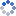加载中…氯化钡和硫酸银
• 博客等级：• 博客积分：0
• 博客访问：91,145
• 关注人气：37
• 获赠金笔：0支
• 赠出金笔：0支
• 荣誉徽章：

## 基础集合论（2）：关系与函数(2015-10-18 14:29:07)

### 杂谈

我们在各种场合经常用到函数，却并没有给函数下一个严谨的定义。“对于集合 X 中任意一个 x，都有集合 Y 中唯一确定的 y 与之对应”，对应是什么东西？唯一确定又怎么表示？
实际上，如果我们定义了有序对的概念，我们可以把所有可能的 (x, f(x)) 拿出来组成一个集合，这样“唯一确定”就可以用“若 (x, y), (x, z) 都在这个集合里，那么 y=z ”来表述。并且更一般地，我们还可以定义“关系”的概念：例如，任取两个实数 x, y，则要么 x 小于 y 要么 x 不小于 y，这时我们把所有满足 x
作为讨论关系与函数的基础，我们先来定义有序对和笛卡尔积的概念。
有序对通常的记法是 (a, b)（当然我们研究的是抽象的集合论，所以毫无疑问 a, b 都是集合），大多数人最先见到的有序对应该是平面直角坐标系的坐标了，此时 (a, b) 中 a, b 都是实数。对于有序对，我们没那么多要求，只要下式成立就够了：看起来很简单，实际操作起来却不容易：我们早就用公理确定了 {a, b} 的存在，可惜这里 {a, b}={b, a}，不满足要求，我们要用一些奇葩的手段把 a, b 区别开（用普通的手段是不行的，比如说，我们可以定义 (a, b)={a, {b}}，但是这样的话会得到 ({a}, b)=({b}, a)={{a}, {b}}，不满足要求）。历史上第一个有序对的定义是 Norbert Wiener 给出的 (a, b):={{{a}, Ø}, {{b}}}，这样 a, b 总算是区别开了。不过，这种定义稍微复杂了一点，现在常用的定义是 Kuratowski 给出的：接下来我们证明上面那条结论正确就行了，也就是说，我们要证明实际上，设左边等式成立，则 {a} 属于等号左边，因此也属于等号右边，即第二行是由第一行用偶集公理推出来的。不管前者还是后者成立，均有 c 属于等号右边，也就是说 c∈{a}，从而 a=c 。代入原式得这样，{a, b} 属于等号左边，因此也属于等号右边，同样用偶集公理得于是 b 属于等号左边，因此 b 要么属于 {a} 要么属于 {a, d}，于是 b=a 或 b=d 。如果 b=d 那么直接得证，否则 b=a，用完全相同的方法可以得出 d=a 或 d=b，不管怎么样都有 b=d 。
这样，我们就证明了：若 (a, b)=(c, d)，则 a=c 且 b=d 。对于任意的 x，如果它是有序对（即存在 a, b 使得 x=(a, b)），那么立即可得 a, b 都是唯一的，我们把 a, b 分别叫做 x 的第一坐标和第二坐标。
紧接着，我们定义笛卡尔积。如果我们最常见的有序对就是平面上点的坐标，那么笛卡尔积就相当于平面上所有点的集合。形式化地说，给定两个集合 A, B，所有满足 a∈A, b∈B 的有序对 (a, b) 组成的集合就是 A, B 的笛卡尔积。不过在此之前，我们先要证明，这是一个集合！集合的条件已经有了：存在 a∈A, b∈B，使得 x=(a, b)，再找一个包容集就行了。
实际上，考虑 (a, b)={{a}, {a, b}}，那么 a, b 均属于 A∪B，于是 {a}, {a, b} 均属于 P(A∪B)，进而 {{a}, {a, b}} 属于 P(P(A∪B))，于是 P(P(A∪B)) 就是我们要找的包容集（注意这里找包容集借助了幂集公理）。由子集公理，下面的集合存在且唯一：我们把它称为 A, B 的笛卡尔积，记作 A×B，上面的集合还可简记为注意，A×B=B×A 不一定成立。
我们的准备工作已经做好，接下来就来定义关系与函数。

关系的定义：一个以有序对为元素的集合称为一个关系。也就是说，若集合 R 满足那么集合 R 是一个关系。通常也把 (a, b)∈R 记作 aRb（把 R 换成小于号，那么 aRb 的记法就舒服多了）。
关系是什么？仍然以小于关系来说，我们最初对小于的叙述是“对任意实数 x, y，x”。利用集合的语言，我们把所有满足 x
不过还好，我们经常只考虑两个集合之间的关系（或者一个集合上的关系），因此我们定义：当集合 R 是 X×Y 的子集时（由于 X×Y 的元素全为有序对，故 R 必为关系），就说 R 是 X 与 Y 的一个关系。特别地，当 R 是 X×X 的子集时，说 R 是 X 中的一个关系。
接下来，我们证明一个结论：对任意关系 R，下面两个集合存在且唯一：实际上，由 {{x}, {x, y}}∈R 得 {x, y}∈∪(R)，进而有 x, y∈∪(∪(R))，故 ∪(∪(R)) 就是这两个集合的包容集，由子集公理，这两个集合存在且唯一。我们把这两个集合分别叫做 R 的定义域和值域（它们分别表示关系 R 元素的两个坐标的取值范围）：显然若 R 是 X 与 Y 的一个关系，则 dom(R), ran(R) 分别是 X, Y 的子集。
最后，我们定义关系的逆与复合：对任意关系 R, S，定义直观地说，关系的逆，就是所有元素的两个坐标交换一下；关系的复合，实际上是关系的传递：若 aRb, bSc 同时成立，那么 a(SR)c 也成立。不过，事情还没完：我们要给这两个集合找一个包容集，来保证它们确实是集合。事实上容易验证，ran(R)×dom(R)，dom(R)×ran(S) 分别是我们要找到的两个包容集。
至于为什么记法中 S, R 顺序颠倒了一下，实际上是遵守了复合函数的习惯：gf(x) 就等于 g(f(x)) 。
关系的逆与复合满足下面的性质：对任意关系 R, S, T，有这些用定义都容易证明。

接下来讨论一种特殊的关系：集合 X 中的关系 R 称为等价关系，当且仅当对任意 x, y, z∈X，满足下面三式：这三条性质分别被称为自反性、对称性、传递性。若 xRy，则称 x, y 等价。对任意集合 X，显然 {(x, x}|x∈X} 是等价关系，同时它被 X 中所有等价关系包容（因为自反性）。由自反性，顺便得到关系 X 的定义域和值域均为 X 本身。另一方面，X×X 本身也是等价关系，同时它包容 X 中的所有等价关系。
对于等价关系，我们定义等价类的概念：x 的 R-等价类定义为也就是所有与 x 等价的 X 中元素的集合。由此，我们立即得到实际上，若 [x]_R=[y]_R，则由自反性 x∈[x]_R=[y]_R，再由等价类定义有 xRy；反过来，若 xRy，那么对任意 z∈X，由自反性 xRz 当且仅当 yRz，即 z 属于 [x]_R 当且仅当 z 属于 [y]_R，再由外延公理得 [x]_R=[y]_R 。
不仅如此，我们可以证明 xRy 不成立当且仅当 [x]_R 与 [y]_R 交集为空，这也就是说实际上，若 [x]_R 交 [y]_R 非空，则存在 z 同时属于二者，由 xRz, yRz 及传递性得 xRy；反过来，若 xRy，那么必有 x 同时属于 [x]_R, [y]_R，故二者交集非空。
综合上面两条性质，立即可得：两个等价类要么完全相等，要么没有任何公共元素。同时我们还知道，任意一个等价类非空（任意 [x]_R 含有元素 x），X 中任意元素都在一个等价类中（任意元素 x 都属于 [x]_R）。这样，我们对于等价类总算有了个清晰的印象：所有等价类（不考虑重复的）两两不相交，并且覆盖了整个集合 X 。
一般地，给定集合 X，若集合 M 的元素全为 X 的非空子集，并且满足下面两式：那么 M 叫做 X 的一个分类。显然根据上面的讨论，给定集合 X 中的一个关系 R，那么所有等价类组成的集合是 X 的一个分类。反过来，若给定 X 上的一个分类 M，那么（即只要 x, y 同属于 M 的一个元素，(x, y) 就属于关系 R）是 X 中的一个关系，并且
1. R 满足自反性：对 X 中任意元素 x，由 x∈X=∪(M) 得存在 M 的元素 A 包含 x，由定义 (x, x)∈R；
2. R 满足对称性：(x, y)∈R 说明存在 M 的元素 A 同时包含 x, y，也就是说 (y, x)∈R；
3. R 满足传递性：(x, y), (y, z)∈R 说明存在 M 的元素 A, B 分别包含 x, y 和 y, z，从而 A, B 交集非空，故由定义 A=B，于是 A 同时包含 x, z，即 (x, z)∈R 。
因此 R 是 X 中的一个等价关系。可以看到，集合 X 的等价关系和划分是密切相关的。给定 X 中的等价关系 R，通常称前面讨论过的等价类组成的集合为 X 的 R-商集，即定义了关系，再定义函数就简单了：函数是一种特殊的关系，对任意的 x，总有至多一个 y 与之对应。换句话说，一个关系 F 若满足下列条件：由此可知，对任意 x∈dom(F)，存在 y 使得 xFy（由定义域的定义），并且这样的 y 是唯一的（由函数的定义）。这时，我们就记 F(x)=y，并称 F(x) 为函数 F 在 x 的值。
若 X 是函数 F 的定义域，而 Y 包容函数 F 的值域，就说 F 是从 X 到 Y 的一个函数，记作 F : X→Y 。
先看一个小问题：给定集合 X, Y，考虑从 X 到 Y 的所有函数，它们能否组成一个集合？这里，集合的条件已经有了，只要找一个包容集就行了。注意到对任意的 F : X→Y，都有 dom(F)=X, ran(F) 包容于 Y，故 F 是 X×Y 的子集，于是 P(X×Y) 就是我们要找的包容集。由子集公理，这样的集合存在且唯一，我们把它记作 Y^X 。
下面是一些简单情况：首先，对任意集合 Y 有注意到 Y^X 是 P(X×Y) 的子集，而 P(Ø×Y)=P(Ø)={Ø}，于是 Y^Ø 是 {Ø} 的子集。另一方面，按定义，显然 Ø 是一个从 Ø 到 Y 的函数，因此 Y^Ø={Ø} 。这是很容易理解的：一个定义域为空集的函数不会有任何元素。
另外，对任意非空集合 X 有这是因为：根据同样的道理，Y^X 是 P(X×Y) 的子集，而 P(X×Ø)=P(Ø)={Ø}，于是 Y^Ø 是 {Ø} 的子集。但是，函数 Ø 的定义域是 Ø 不等于 X 。因此 Ø 不属于 Y^Ø，Y^Ø 是空集。这个也容易理解：对于 X 中的元素必然有 Y 中的元素对应，然而这里 X 的元素找不到任何 Y 的元素对应，因此这种对应是不可能存在的。
接下来定义一些常用的概念：若对任意的 y，至多有一个 x 使得 y=F(x)，也就是说对于函数 F : X→Y，若 Y=ran(F)，则称 F 为满射（这里要指明 F 从哪个集合到哪个集合，否则 F 总是 dom(F) 到 ran(F) 的满射，这个定义就成废话了。如果 F : X→Y 既是单射又是满射，就称该函数为双射。
对于函数 F 及 dom(F) 的一个子集 A，记最后，定义象和原象的概念：设 F : X→Y，A 是 X 的一个子集，记类似地，设 F : X→Y，B 是 Y 的一个子集（注意，B 不一定包容于 ran(F)），记下面两条性质很常用：设 A, B 分别为 X, Y 的子集，则证明第一式：任取 A 中元素 x，由定义 F(x) 属于 F(A)，再对照原象的定义，x 必为 F^(-1)(F(A)) 的元素。若 F 为单射，则任取 F^(-1)(F(A)) 中元素 x，有 F(x) 属于 F(A)，根据象的定义，必存在 t∈A 使得 F(t)=F(x)，从而 t=x∈A，故此时包容号可以改为等号。
类似地证明第二式：任取 F(F^(-1)(B)) 中元素 y，必有 x∈F^(-1)(B) 使得 y=F(x)，而 x∈F^(-1)(B) 说明 F(x)∈B，也就是 y∈B 。若 F 为满射，则任取 B 中元素 y，必存在 x∈X 使得 y=F(x)，由 F(x)∈B 得 x∈F^(-1)(B)，进而 y=F(x)∈F(F^(-1)(B))，故此时包容号可以改为等号。
将关系的逆与复合推广到函数上，可以定义反函数与复合函数的概念。关系的逆一定是关系，但函数的逆却不一定是函数，我们有定理：对于函数 F，F^(-1) 为函数当且仅当 F 是单射。这件事很容易证明（看一下函数定义及单射定义，它们正好相反），于是当 F^(-1) 是函数时，我们说 F^(-1) 是 F 的反函数。
容易证明，单射 F 的反函数也是单射，并且 F 的反函数的反函数是 F 本身。若 F : X→Y 是双射，则 F^(-1) 是从 Y 到 X 的双射。下面两式也容易证明：关系与关系复合仍然是关系，函数与函数复合还是不是函数？答案是肯定的，我们现在就来证明：若 F, G 是函数，则 GF 仍然是函数。证明的基本工具是定义，为此我们先设根据复合关系的定义，存在 y_1, y_2 使得由 F 是函数得 y_1=y_2，再由 G 是函数得 z_1=z_2，从而 GF 是函数。
GF 的定义域是什么？用直觉考虑一下，若 x 在 GF 的定义域内，首先要有 x 属于 dom(F)，其次 F(x) 要属于 dom(G) 。后一条实际上就是 x 属于 F^(-1)(dom(G))，因此我们推出正式的推理如下：因为复合函数是复合关系的特殊情况，所以我们立即有：对函数 F, G, H，有关于复合的另一条性质与逆有关，因此有了前提条件：对单射 F, G，有只需证明 GF 也是单射即可，证明与刚才证 GF 是函数一样。

在本文的最后，引入集族的概念。实际上，集族就是一个函数，函数的定义域叫做标集，标集的元素叫做标号，函数值叫做集族的项。为了说清楚这些名词的来历，先举个例子：有时我们需要考虑一串集合 A_0, A_1, A_2, ...，就可以定义一个定义域为自然数集（对自然数集的定义，将在下篇文章中叙述）的函数 F，标集就是自然数集，标集的元素 0, 1, 2, ... 叫做标号，而 F(0), F(1), F(2), ... 叫做项，我们把它记作 A_0, A_1, A_2, ... 。这些名词与数列中用的名词是类似的。
一般地，我们用有了集族这个工具，先把并集交集推广到集族上：注意，第二个定义要求标集 I 非空，否则无意义。为了保证这两个集合存在，只需注意到：设 M 为该集族（函数）的值域，则二者分别等于 ∪(M) 和 ∩(M) 。
现在我们讨论并（交）集的结合律的问题。所谓结合律，就是先把集族的元素分组，每组分别求并（交）集，然后所有结果再求并（交）集，结果与直接求交（并）相同。把集族的元素分组，实际上是把标集 I 分组，为此我们要设出一个新的集族，它的元素都是标集 I 的子集，并且并集就是标集 I（至于它们是不是两两不交，我们不关心）。也就是说，设其中 K 是新的集族 (J_k)_(k∈K) 的标集。这样，我们就可以写出结合律的式子了：证明这两个式子不难。注意，第二个式子要求 I, K 以及每个 J_k 都非空。
我们之前只对两个集合定义了笛卡尔积，现在我们可以在集族上定义笛卡尔积。给定集族 (A_i)_(i∈I)，从每个集合 A_i 中取出一个元素 a_i，构成一个集族 (a_i)_(i∈I)，所有这样的集族组成的集合就称为集族 (A_i)_(i∈I) 的笛卡尔积。问题是，它是不是一个集合？注意到，任意取出的 (a_i)_(i∈I) 总是一个定义在 I 上的函数，每个函数值 a_i 都属于 A_i，于是也属于集族 (A_i)_(i∈I) 的并（记为 M），这样 M^I 就是我们要找的包容集。
接下来是正式的定义：我们记0